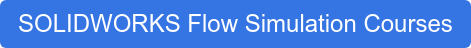Symmetry and periodic boundary conditions in SOLIDWORKS Flow Simulation are used to reduce the size of the flow problem, thereby reducing analysis run time. Although these two computational domain boundary conditions are set up by editing the default computational domain, they both are very different.

#### Symmetry Boundary Condition

If we know for certain that an internal or external flow contains one or more symmetry planes, we can resize the computational domain to a relevant flow region. Use of symmetry in Flow Simulation can be a challenge because the flow field may not always be symmetric, even if the boundary condition and geometry are symmetric. For example, the Von Kármán vortex street (vortex shedding) for flow past a cylinder has unsteady flow separation seen at high velocities or swirling flow. Using symmetry boundary condition in such scenarios will lead to an incorrect solution as the flow will not be along a symmetry plane. To use symmetry, either axial or planar, we must take into consideration not only the symmetry of geometry and boundary condition but also the flow field.

The image below shows an example of a symmetry boundary condition where the computation domain has symmetry defined in global Y and Z direction.#### Periodic Boundary Condition

Unlike symmetric boundary, the periodic boundary condition can be used to analyze fluid flow in models consisting of identical geometrical features arranged in a periodic linear order, for example, a linear pattern or an array of identical fins or PCBs in an enclosure. For such geometries, we can specify the periodicity boundary condition at the domain boundaries, along with the direction of the linearly patterned geometrical feature.

#### Repeating Geometric Patterns

It's important to note that periodicity cannot be used to simulate a repeating geometric pattern where the inlet/outlet condition of one repeated instance becomes outlet/inlet of the next period. We can define periodic boundary conditions for only two pairs of boundaries, for example, for two directions or use it for one pair of boundaries along with symmetry for another pair. In both cases, the third pair of boundaries must have the default settings.

In the image below, the periodicity boundary condition is defined for one pair of boundaries, in this case, the Z direction. The periodic or repeating regions are indicated by the dotted lines around the computational domain.#### Axial Periodicity

The axial periodicity option introduced in SOLIDWORKS Flow Simulation 2018 can be used to analyze fluid flow in models, for example, an impeller. Axial periodicity option saves analysis time as fluid flow is calculated only for a sector instead of the entire 360 degrees of an asymmetric model. This can be used to simulate problems within a sector such as a rotating region and/or heat conduction in solid. Phase transitions, free surface, porous media, high Mach number flow and local region rotation of averaging type are not yet allowed for this option.The image below defines the parameter inputs for axial periodicity as defined in Flow Simulation help topics.#### Defining Sectors for Axial Periodicity

When defining sectors for axial periodicity, the location of geometry within the sector of periodicity should not matter. For example, in the case of an impeller with highly curved blades, it would be okay if the blade geometry crosses the boundary of a sector and doesn’t completely lie within the sector.

To verify this, I analyzed an impeller model to evaluate its efficiency. Three different flow configurations were analyzed. The original flow project used the full impeller model. Two projects cloned off the original one and used axial periodicity option with varying sector angle to enclose different regions of blade geometry. As shown in the image below, efficiency evaluated for both axial periodicity projects were similar and matched closely to the original project as expected.Using the technique detailed above, flow simulation users can reduce the design validation time and speed up the overall product development process.

Interested in learning more about SOLIDWORKS Flow Simulation? Check out our upcoming training courses.